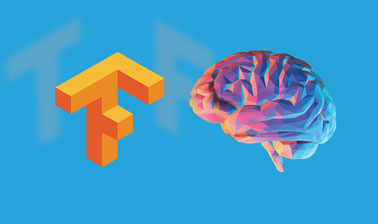Deep Learning with Tensorflow

Learn how to apply Deep Learning with TensorFlow to data to solve real-world problems.Free

##### Description

Featured on: Oct 12, 2018

Traditional neural networks rely on shallow nets, composed of one input, one hidden layer and one output layer. Deep-learning networks are distinguished from these ordinary neural networks having more hidden layers, or so-called more depth. These kind of nets are capable of discovering hidden structures within unlabeled and unstructured data (i.e. images, sound, and text), which consitutes the vast majority of data in the world.TensorFlow is one of the best libraries to implement deep learning. TensorFlow is a software library for numerical computation of mathematical expressional, using data flow graphs. Nodes in the graph represent mathematical operations, while the edges represent the multidimensional data arrays (tensors) that flow between them. It was created by Google and tailored for Machine Learning. In fact, it is being widely used to develop solutions with Deep Learning.In this TensorFlow course, you will learn the basic concepts of TensorFlow, the main functions, operations and the execution pipeline. Starting with a simple “Hello Word” example, throughout the course you will be able to see how TensorFlow can be used in curve fitting, regression, classification and minimization of error functions. This concept is then explored in the Deep Learning world. You will learn how to apply TensorFlow for backpropagation to tune the weights and biases while the Neural Networks are being trained. Finally, the course covers different types of Deep Architectures, such as Convolutional Networks, Recurrent Networks and Autoencoders.

Free